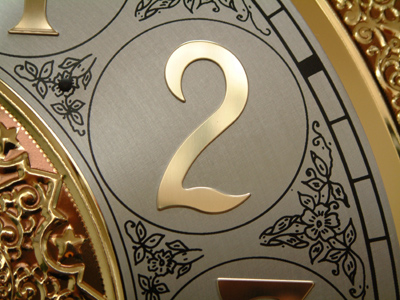What is 16 x 8 as a power of 2?

# Level 5-6 Numbers - Powers

Powers, indices, exponents. Three words which may worry you but you need to understand them for KS3 Maths. So, what exactly are they? Well, all three terms mean exactly the same thing. They refer to the little numbers placed above and to the right of ordinary numbers. These little numbers, most commonly referred to as powers, tell you to multiply the large number by itself as many times as the power tells you.

Squares and cubes are the powers you'll come across most often. They're simply a way of quickly writing a lot of information. For example 47 is much easier to write than 4 x 4 x 4 x 4 x 4 x 4 x 4 but means exactly the same thing.

Raising a number to a power is a POWERFUL operation, that's why I for Index comes before DM for Division and Multiplication in the order of operations you know as BIDMAS. Try this quiz to help you practise your powers in maths!

1.
What is 32 x 22 as a single power number?
64
62
54
none of these
3 x 3 x 2 x 2 = (3 x 2) x (3 x 2)
2.
What is 16 x 8 as a power of 2?
25
26
27
28
16 = 24; 8 = 23. To multiply powers of the same number you add the indices
3.
What is 83 as a single power of 2?
26
27
28
29
83 = (2 x 2 x 2)3 = (23)3. To raise a power to a power, multiply the indices
4.
What is 54 ÷ 52?
5
25
125
625
To divide powers of the same base you subtract the indices; 5(4 - 2) = 52
5.
What is 43 x 34 as a single power number?
127
712
77
none of these
You can't add the indices to multiply unless both numbers are powers of the same base
6.
Which of these gives the biggest number?
28
82
36
63
It's not always obvious which power number is the largest. The best way to check is to use a calculator!
7.
What is one hundred million as a power of 10?
105
106
107
108
The power of ten is the same as the number of zeroes. 100,000,000 has 8 noughts
8.
What is the value of 24?
8
16
32
64
24 (two to the power four) = 2 x 2 x 2 x 2
9.
Which of these is not the same as 92 x 81?
94
38
89
6,561
9 x 9 x 9 x 9
10.
What is the value of 2-3?
1/3
1/6
1/8
1/9
A negative power is 1 divided by the positive power